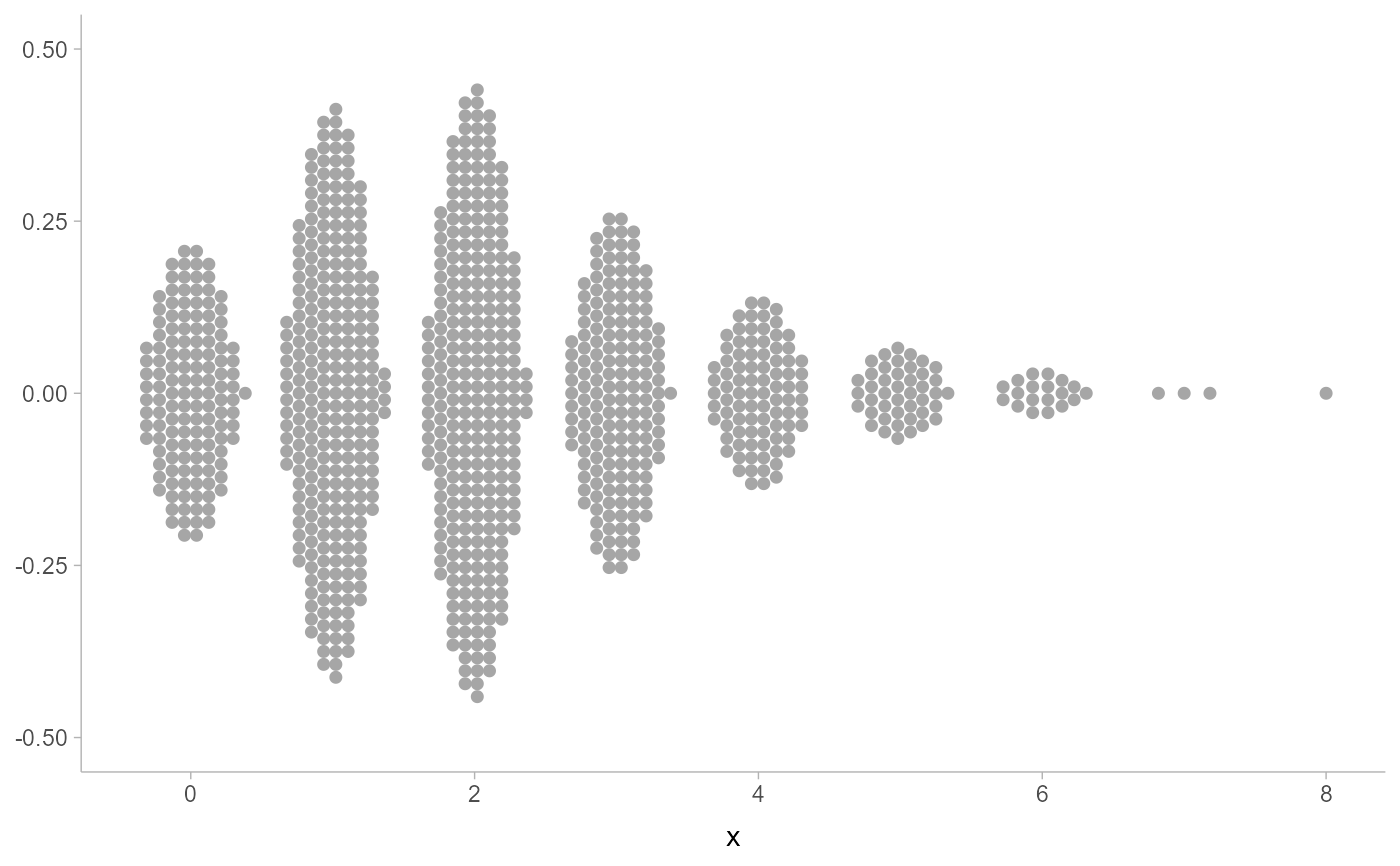Note: Better-looking bar dotplots are typically easier to achieve using layout = "bar" with the geom_dotsinterval() family instead of smooth = "bar" or smooth = "discrete".

Smooths x values where x is presumed to be discrete, returning a new x of the same length. Both smooth_discrete() and smooth_bar() use the resolution() of the data to apply smoothing around unique values in the dataset; smooth_discrete() uses a kernel density estimator and smooth_bar() places values in an evenly-spaced grid. Can be used with a dotplot (e.g. geom_dots(smooth = ...)) to create "bar dotplots". Supports automatic partial function application.

smooth_discrete(
x,
kernel = c("rectangular", "gaussian", "epanechnikov", "triangular", "biweight",
"cosine", "optcosine"),
width = 0.7,
...
)

smooth_bar(x, width = 0.7, ...)

## Arguments

x

a numeric vector

kernel

string: the smoothing kernel to be used. This must partially match one of "gaussian", "rectangular", "triangular", "epanechnikov", "biweight", "cosine", or "optcosine". See stats::density().

width

approximate width of the bars as a fraction of data resolution().

...

additional parameters; smooth_discrete() passes these to smooth_unbounded() and thereby to density_unbounded(); smooth_bar() ignores them.

## Value

A numeric vector of length(x), where each entry is a smoothed version of the corresponding entry in x.

If x is missing, returns a partial application of itself. See automatic-partial-functions.

## Details

smooth_discrete() applies a kernel density estimator (default: rectangular) to x. It automatically sets the bandwidth to be such that the kernel's width (for each kernel type) is approximately width times the resolution() of the data. This means it essentially creates smoothed bins around each unique value. It calls down to smooth_unbounded().

smooth_bar() generates an evenly-spaced grid of values spanning +/- width/2 around each unique value in x.

Other dotplot smooths: smooth_density, smooth_none()

## Examples


library(ggplot2)

set.seed(1234)
x = rpois(1000, 2)

# automatic binwidth in basic dotplot on large counts in discrete
# distributions is very small
ggplot(data.frame(x), aes(x)) +
geom_dots()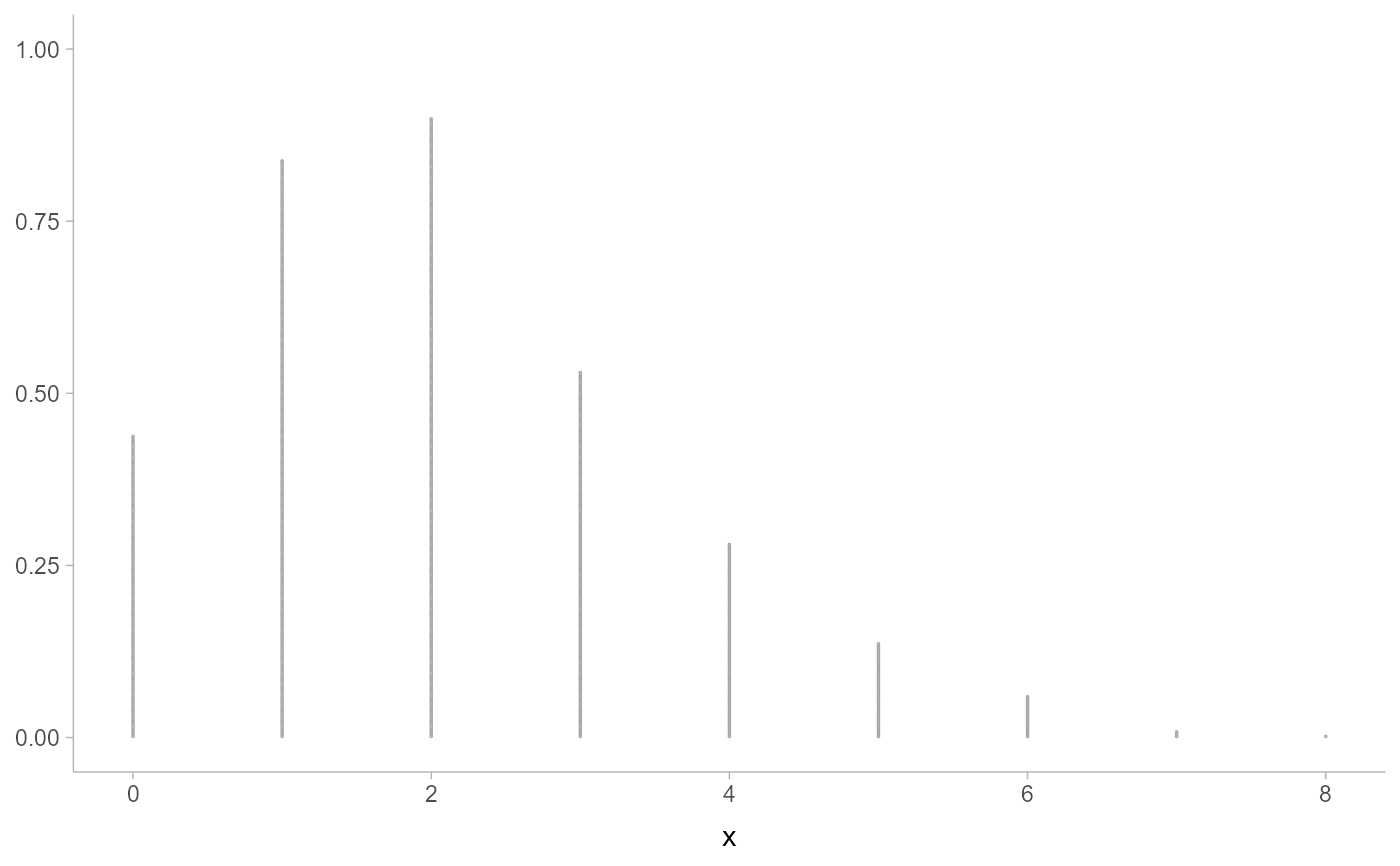# NOTE: It is now recommended to use layout = "bar" instead of
# smooth = "discrete" or smooth = "bar"; the latter are retained because
# they can sometimes be useful in combination with other layouts for
# more specialized (but finicky) applications.
ggplot(data.frame(x), aes(x)) +
geom_dots(layout = "bar")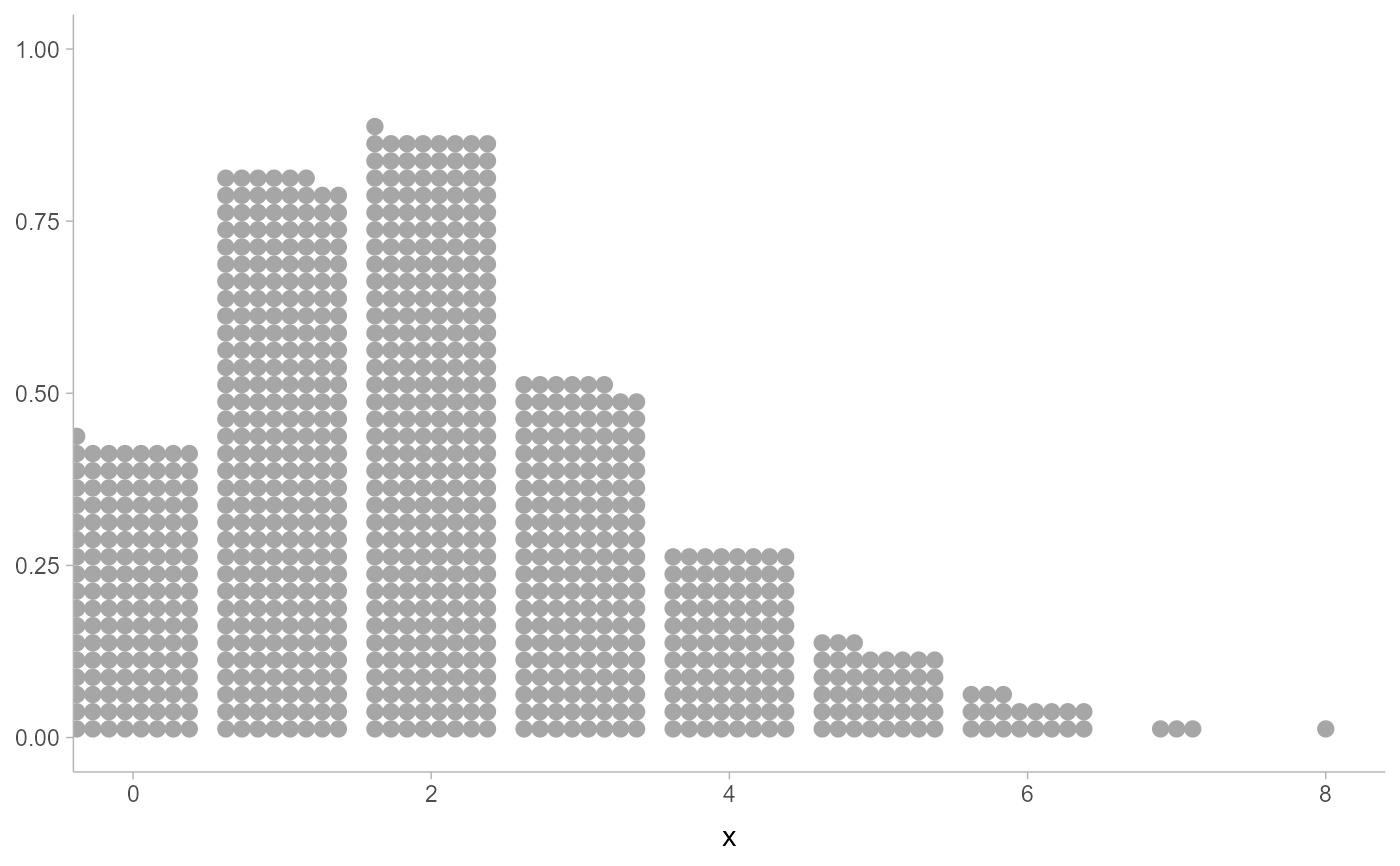# smooth_discrete() constructs wider bins of dots
ggplot(data.frame(x), aes(x)) +
geom_dots(smooth = "discrete")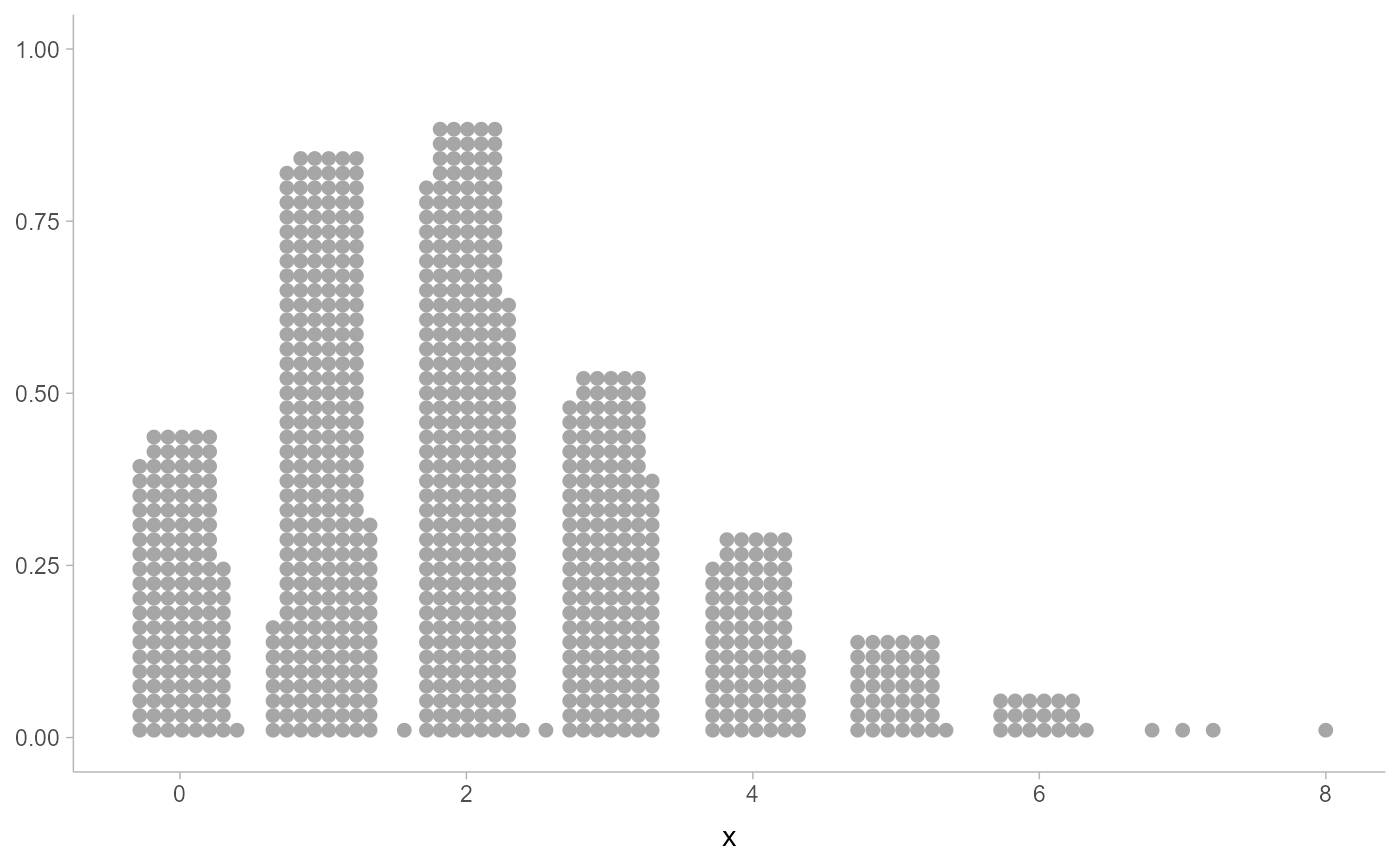# smooth_bar() is an alternative approach to rectangular layouts
ggplot(data.frame(x), aes(x)) +
geom_dots(smooth = "bar")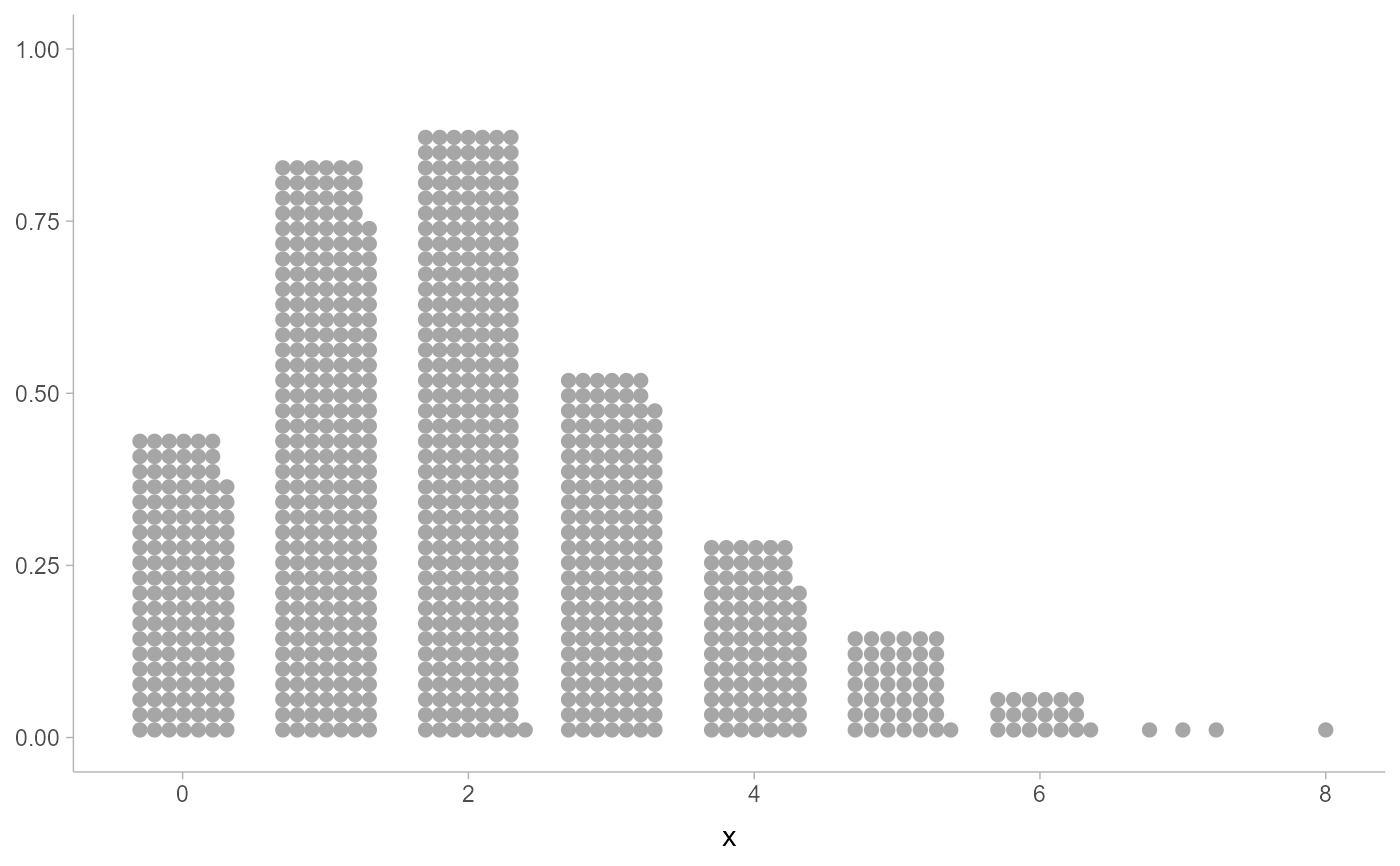# adjust the shape by changing the kernel or the width. epanechnikov
# works well with side = "both"
ggplot(data.frame(x), aes(x)) +
geom_dots(smooth = smooth_discrete(kernel = "epanechnikov", width = 0.8), side = "both")